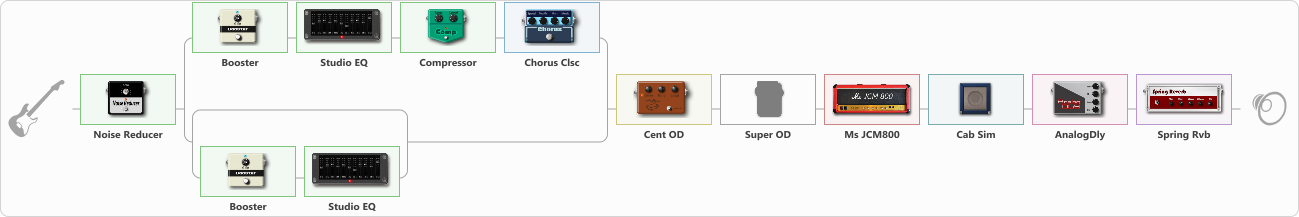# Blues cruch tone truss

Discussion in 'ToneLib-GFX presets' started by truss van halen, Oct 26, 2020.

1. ### truss van halenWell-Known Member

Blues cruch tone truss

Preset name: Blues cruch tone truss

Jackson soloist II

Effects chain:Effect: "Noise Reducer" (Dynamics / Filter), active - "yes"
{
"Sens" = 88
"Mode" = Hard
}

Effect: "Splitter" (Dynamics / Filter), active - "yes"
{
"A-Bypass" = Off
"A-Pan" = 0
"A-Level" = 55
"B-Bypass" = Off
"B-Pan" = 0
"B-Level" = 55

'A' branch:
{

Effect: "Booster" (Dynamics / Filter), active - "yes"
{
"Gain" = 71
}

Effect: "Studio EQ" (Dynamics / Filter), active - "yes"
{
"31 Hz" = 0
"62 Hz" = 0
"125 Hz" = 0
"250 Hz" = 0
"500 Hz" = 0
"1 kHz" = 0
"2 kHz" = 0
"4 kHz" = 0
"8 kHz" = 0
"16 kHz" = 0
"Level (dB)" = 0
}

Effect: "Compressor" (Dynamics / Filter), active - "yes"
{
"Sense" = 21
"Level" = 70
}

Effect: "Chorus Clsc" (Modulation / Sfx), active - "yes"
{
"Speed" = 3.5
"Depth" = 58
"Center" = 1.0
"Mode" = Stereo
}
}
'B' branch:
{

Effect: "Splitter" (Dynamics / Filter), active - "yes"
{
"A-Bypass" = Off
"A-Pan" = 0
"A-Level" = 55
"B-Bypass" = Off
"B-Pan" = 0
"B-Level" = 55

'A' branch:
{
}
'B' branch:
{

Effect: "Booster" (Dynamics / Filter), active - "yes"
{
"Gain" = 25
}

Effect: "Studio EQ" (Dynamics / Filter), active - "yes"
{
"31 Hz" = 0
"62 Hz" = 0
"125 Hz" = 0
"250 Hz" = 3
"500 Hz" = 5
"1 kHz" = 0
"2 kHz" = 0
"4 kHz" = -2
"8 kHz" = 0
"16 kHz" = 0
"Level (dB)" = 5
}
}
}
}
}

Effect: "Cent OD" (Overdrive / Distortion), active - "yes"
{
"Drive" = 12
"Tone" = 76
"Level" = 38
}

Effect: "Super OD" (Overdrive / Distortion), active - "no"
{
"Drive" = 0
"Tone" = 80
"Level" = 91
}

Effect: "Ms JCM800" (Amp simulators), active - "yes"
{
"Gain" = 50
"Bass" = 27
"Middle" = 70
"Treble" = 84
"Presence" = 50
"Master" = 50
"Level (dB)" = 0
}

Effect: "Cab Sim" (Cabinets), active - "yes"
{
"Model" = 1x12" Blue Bell
"Level (dB)" = 0
}

Effect: "AnalogDly" (Delay), active - "yes"
{
"Time" = 532
"Feedback" = 26
"Tone" = 55
"Mix" = 55
}

Effect: "Spring Rvb" (Reverberation), active - "yes"
{
"Time" = 3.3
"PreDelay" = 0
"LoDamp" = 16
"HiDamp" = 26
"Mix" = 42
}

Note: You will need to download and install the ToneLib-GFX software to use the preset.

#### Attached Files:

• ###### Blues_cruch_tone_truss.tlgfx
File size:
1.3 KB
Views:
2,524
Sergejs Matvejenkovs likes this.